# MTPS FAQ:  How Does Thermal Radiation Affect Thermal Conductivity Measurement

### Part I: Opaque Samples

Recently, a researcher asked how the Modified Transient Plane Source method accounts for the problem of thermal emissivity. Given that most thermal conductivity measurement methods need to control for – and, ideally, eliminate – heat transfer methods other than thermal conduction, controlling the effect of thermal radiation is a valid concern for anyone interested in thermophysical properties analysis methods, we thought it may be wise to address the issue in a blog post.

Per the Stefan-Boltzmann law, radiation is emitted by every material, at all times, according to the following equation: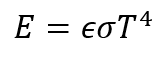… where E is the energy flux per unit surface area, ϵ is the emissivity of the material, ϵ is the Stephan-Boltzmann constant, and T is the temperature in an absolute scale (Kelvin for SI units, Rankine if you’re using US units).  An obvious consequence of this equation is that the thermal radiation from a material to its surroundings by thermal radiation is proportional to the fourth power of the absolute temperature – thus, radiation increasingly becomes a dominant mode of heat transfer as temperature increases.

Considering heat transfer between two materials (A and B) at different temperatures (Tand TB), the radiation is governed by the difference in the two energy fluxes, the effective emissivity, a geometric shape factor, which accounts for difference in material geometries and orientation, and the effective surface area: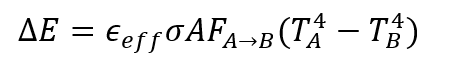In the case of two materials which are in extremely close proximity (as with the sample and sensor), and considering the two of them in isolation, the shape factor may be assumed to be 1, so it simplifies: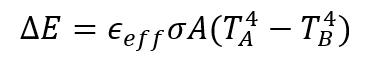Then we can also assume, just to obtain a “worst case scenario” model, that both materials behave as black bodies. In this case, the emissivity becomes 1 and the equation simplifies further.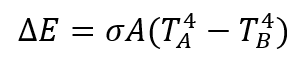A consequence of this equation is that if each material is at the same temperature, the difference reduces to 0 and the net heat transfer is nil. If the interface of two materials in intimate thermal contact, each material at the interface is at the same temperature (furthermore, this temperature is governed by the thermal effusivity of each material, if the materials are sufficiently large to behave as semi-infinite media). 

In the case of a test employing a contact agent, the sensor is in perfect thermal contact with the contact agent, which in turn is in perfect thermal contact with the sample, because of the physical nature of fluids. Therefore, fundamentally, radiation is a non-issue in the case of those tests which employ a contact agent (particularly when you consider that the wavelength of black-body radiation at room temperature is roughly 10 micrometers, at which wavelength common contact agents of choice are, in effect, opaque, with absorbance values in the region of 105 or thereabout). The sensor body is constructed to have a large volumetric heat capacity, and its surroundings are kept in thermal equilibrium with the body of the sensor – again, the temperature difference disappears and the net heat transfer is nil. For this reason, for any test involving a contact agent, radiation may safely be neglected.

Now to consider a case which does not have a contact agent. In this case, it’s a bit more complex. A lack of contact agent inherently means there is not perfect thermal contact – there will be gaps between the sensor and the material (the exception here is in the case of liquid samples, which attain perfect contact as detailed above). For simplicity, a series of assumptions may be made, designed to inflate the possible effect of radiation. If radiation is insignificant even in this simplistic worst-case scenario, it is then safely negligible in a real-world scenario where it would certainly play a much smaller role in the overall heat transfer.

-  The previous assumption of black body behavior is again made. This will inflate the heat flux by radiation by setting the effective emissivity as 1.

-  As well, the shape factor is treated as negligible as before. As the sample and sensor are essentially almost touching and their areas essentially completely overlap, this is a reasonable assumption.

-  The volumetric heat capacity of the sensor is again assumed to be sufficiently large that the non-active area of the sensor is again assumed to be isothermal and in thermal with its surroundings – given the small heat flux applied to the sensor and the fact that the sensor body is constructed of stainless steel, which is well-known for its high volumetric heat capacity, this is a reasonable assumption.

-  We assume the maximum roughness of the sample is similar to the maximum roughness of the sensor (10µm), which gives a maximum separation distance of 20µm. This is a reasonable assumption for the majority of test samples.

-  We assume that the maximum roughness is representative of the average separation between the materials – as with the assumption of black body behavior, this is something that is obviously invalid (given that there will be some parts of the sample in intimate contact and if the sample is flexible or deformable, it may behave very closely to something which is in intimate contact with the sensor) but since this has the effect of neglecting the regions in which contact is very good or even intimate, it gives a good worst case scenario for this thought experiment.

-  We will also assume that both materials are black bodies (although this assumption is fairly valid for the sensor surface owing to its glass glaze – glass has an ϵ of around 0.9 – it is certainly invalid for some materials, but this will inflate the effect of radiation and is consistent with the examination of the worst case scenario).

-  We assume that the temperature rise at the sensor during a measurement represents the temperature difference between sensor and sample. For clarity, this is certain to not be the case,  but again, we want to look at the worst-case scenario in this situation. The actual difference in temperature between sample and sensor is difficult to measure but thought to be on the order of a small fraction of a degree.

The overall quantity of energy transferred by radiation between sensor and sample in this worst-case scenario will be 0.0023 W.  This is <1% of the heater power. At 200°C, this worst-case-scenario heat transfer by radiation is 0.012 W – or < 2.5% of the heater power.

Even in this worst-case-scenario at 200°C, radiation is not significant to the measurement. Add in the fact that in such a case, the sensor calibration would compensate to some degree for the effect of emissivity (given that the T rise stays roughly the same at the sensor and the sensor is calibrated to samples of known K value), the fact that it neglects regions of good thermal contact between the sample and the sensor surface, and the fact that this is a gross overestimation of the actual temperature difference between sample and sensor, and we are very confident, both theoretically and in terms of known performance, that emissivity is not a significant confounder to the performance of the sensor at room temperature.

Returning above to the case of a sample with a contact agent, we can verify the assumption that the heat transfer is nil through the water layer:

The following values are necessary to the calculation:

-  Sensor power: 0.5 W

-  Sensor area: 0.000254 m2 (circle with a diameter of 18mm)

-  Maximum possible heat flux through the sensor surface is then: 2000 W/m2

-  The thermal resistance of a 20µm water layer: 3.2 x 10-5 Km2/W

-  Time for the heat wave to cross the water layer: 7 x 10-4 s

The temperature drop across the water layer is then: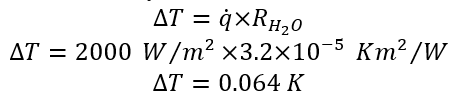Even if we take worst case of all numbers, and assume worst case of discontinuity in temperature between the sensor and sample surfaces, we can only get 0.064K. Using this ∆T as TA-TB in the radiation equation, one can calculate around room temperature (22°C), a maximum heat transfer by radiation of 9.5x10-5W – less than 0.02% of the heat power applied to the sample. There is practically no heat transfer by radiation. At an elevated temperature (e.g., 200°C), the thermal resistance using Wakefield w120 thermal joint compound as a contact agent is 1.5x10-5 Km2/W. The maximum temperature difference, assuming total transparency of the Wakefield compound then becomes 0.029K. The maximum heat transfer by radiation then becomes 1.8x10-4W – less than 0.04% of the total heat power applied to the sample. Again, there is practically no net heat transfer by radiation.

In short: The problem of radiation is something that a lot of consideration has been devoted to – and it can safely be neglected at ordinary operating temperatures of the sensor, even in the worst-case-scenario of no areas of good contact, black-body behavior by sensor and sample, and extremely large temperature, for the purposes of the measurement, difference between sample and sensor.

### Part II: Special Considerations for Glasses and Other Transparent Samples

Previously, we conducted a thought experiment to examine the effect of thermal radiation on an MTPS measurement, looking at the sample and sensor. The question has since been regarding what should be done for transparent and semi-transparent samples like glasses and quartz. In the case of common glasses, the transmission spectra of the materials may be seen below: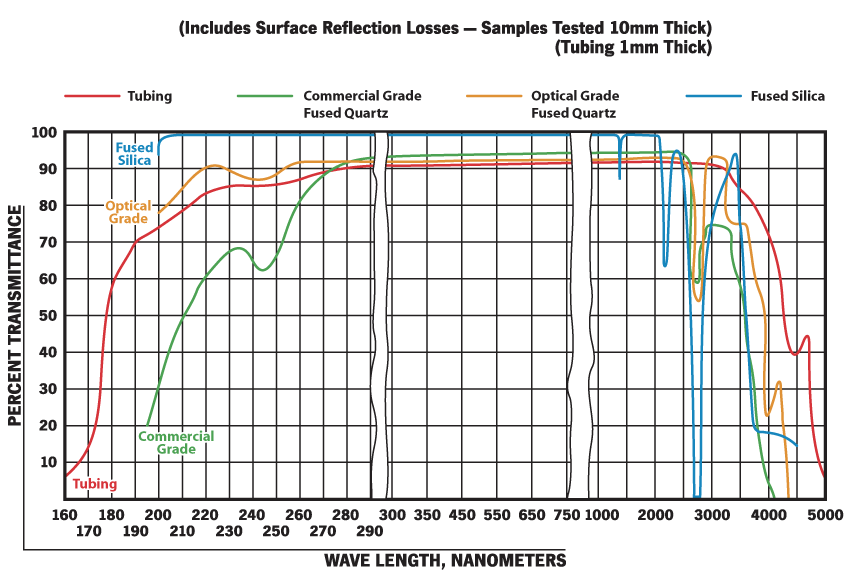Figure 1. Transmittance spectrum of common glasses. (Source)

As can be seen above, most common glasses are essentially opaque to IR radiation beyond a wavelength of 4 µm. The black-body emission spectrum of a material near room temperature may be seen below: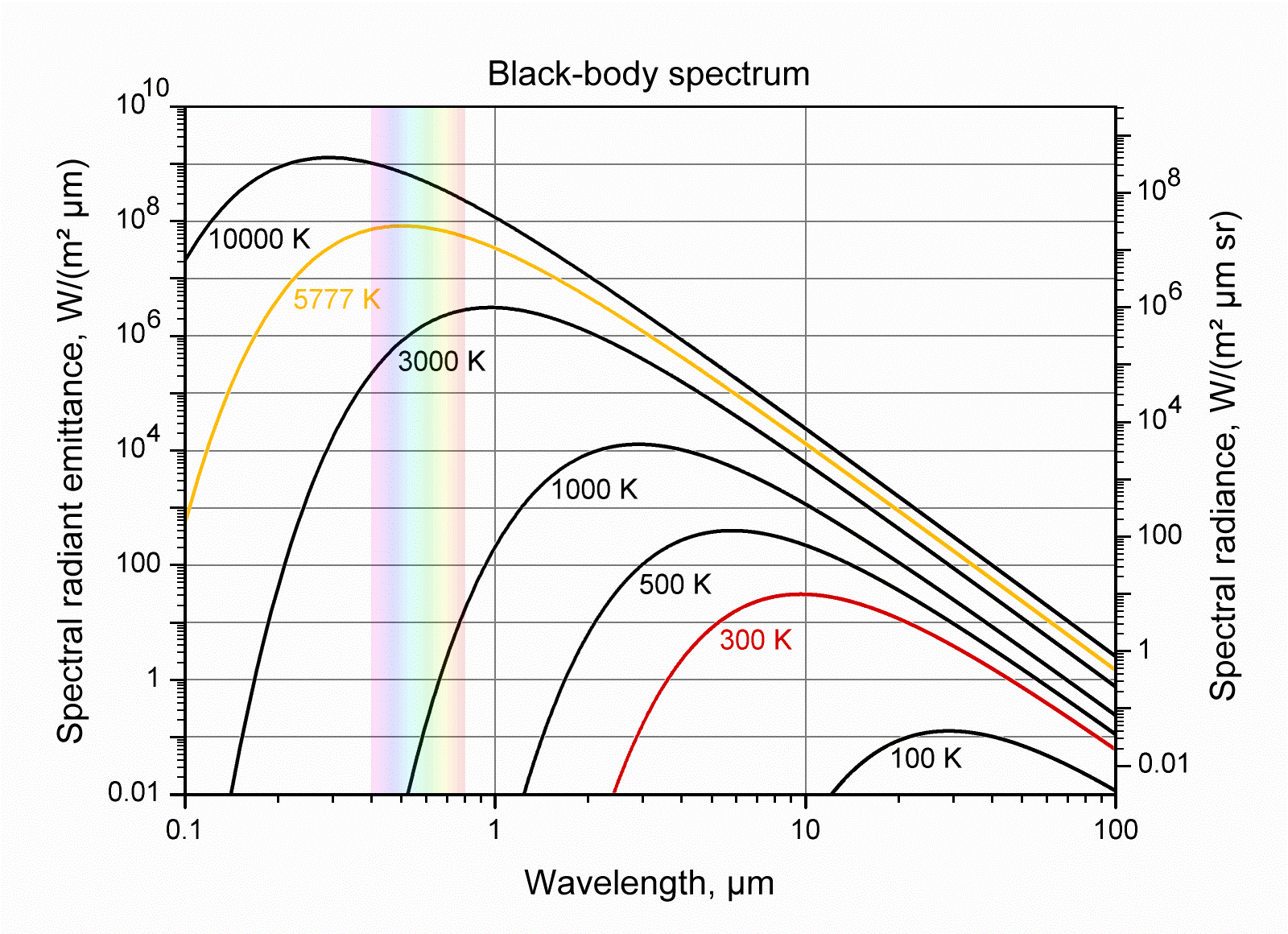Figure 2. Black-body emission spectra at different temperatures. (Source)

It can be seen above that for operational temperatures, the bulk of the thermal radiation incident to a glass sample is at wavelengths greater than 4µm – that is, glass absorbs the light in that wavelength and is effectively opaque. This is the physical reason behind why the thermal emissivity value of glasses, in general, is so high (>0.8).

We’ve seen previously that even in a worst-case scenario, the heat transfer by radiation will not significantly impact the measurement. As glass does not permit the transfer of significant thermal radiation, the effect of thermal radiation in the measurement of glasses and other samples transparent to visible light will not be an issue in typical application of the technique. Thermal radiation may safely be neglected.

 The issues of how one determines effective area, effective emissivity, and shape factor are all worthy topics for consideration – but ultimately beyond the scope of this post. Books can be, and have been, devoted to the subject of heat transfer via thermal radiation. For a more thorough treatment, the reader is recommended to any heat transfer physics textbook, such as A. Mills. Heat Transfer. 1992. Richard D. Irwin, Inc. Burr Ridge, IL. Chapter 6, 487-594, or Howell, Menguc, and Siegel’s Thermal Radiation Heat Transfer, 5th Ed. CRC Press.
 This is governed by the following equation: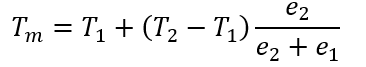Where Tm is the interfacial temperature, T1 and T2 are the bulk temperatures of materials 1 and 2, respectively, e1 ­represents the thermal effusivity of material 1 and e2 represents the thermal effusivity of material 2.
 The reason why this is not the case – and therefore, this assumption grossly inflates any possible effect of radiation – is twofold:
Firstly, the temperature rise at the sensor occurs over a period of 1-3s – thus, the overall temperature difference would become a time dependent function and the possible thermal radiation would then become the integral of this time dependent function.
Secondly, this assumption would require that the sample is isothermal for the duration of the heat pulse. The fact that the sensor is physically able to detect differences in thermal effusivity of materials inherently means that the sample is not isothermal for the duration of the measurement